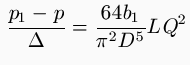[Footnote: A paper lately read before the Institution of Mechanical Engineers.]

By ARTHUR ACHARD, of Geneva.

But allowing that the figure of 22 H. P., assumed for this power (the result in calculating the work with compressed air being 19 H. P.) may be somewhat incorrect, it is unlikely that this error can be so large that its correction could reduce the efficiency below 80 per cent. Messrs. Sautter and Lemonnier, who construct a number of compressors, on being consulted by the author, have written to say that they always confined themselves in estimating the power stored in the compressed air, and had never measured the gross power expended. Compressed air in passing along the pipe, assumed to be horizontal, which conveys it from the place of production to the place where it is to be used, experiences by friction a diminution of pressure, which represents a reduction in the mechanical power stored up, and consequently a loss of efficiency.

The loss of pressure in question can only be calculated conveniently on the hypothesis that it is very small, and the general formula,, is employed for the purpose, where D is the diameter of the pipe, assumed to be uniform, L the length of the pipe, p the pressure at the entrance, p the pressure at the farther end, u the velocity at which the compressed air travels, Δ its specific weight, and f(u) the friction per unit of length. In proportion as the air loses pressure its speed increases, while its specific weight diminishes; but the variations in pressure are assumed to be so small that u and Δ may be considered constant. As regards the quantity f(u), or the friction per unit of length, the natural law which regulates it is not known, audit can only be expressed by some empirical formula, which, while according sufficiently nearly with the facts, is suited for calculation. For this purpose the binomial formula, au + bu², or the simple formula, b u², is generally adopted; a b and b being coefficients deduced from experiment. The values, however, which are to be given to these coefficients are not constant, for they vary with the diameter of the pipe, and in particular, contrary to formerly received ideas, they vary according to its internal surface. The uncertainty in this respect is so great that it is not worth while, with a view to accuracy, to relinquish the great convenience which the simple formula, b u², offers. It would be better from this point of view to endeavor, as has been suggested, to render this formula more exact by the substitution of a fractional power in the place of the square, rather than to go through the long calculations necessitated by the use of the binomial au + bu². Accordingly, making use of the formula b u², the above equation becomes,; or, introducing the discharge per second, Q, which is the usual figure supplied, and which is connected with the velocity by the relation,, we have. Generally the pressure, p, at the entrance is known, and the pressure, p, has to be found; it is then from p that the values of Q and Δ are calculated. In experiments where p and p are measured directly, in order to arrive at the value of the coefficient b, Q and Δ would be calculated for the mean pressure ½(p + p). The values given to the coefficient b vary considerably, because, as stated above, it varies with the diameter, and also with the nature of the material of the pipe. It is generally admitted that it is independent of the pressure, and it is probable that within certain limits of pressure this hypothesis is in accordance with the truth.

D'Aubuisson gives for this case, in his Traité d'Hydraulique, a rather complicated formula, containing a constant deduced from experiment, whose value, according to a calculation made by the author, is approximately b = 0.0003. This constant was determined by taking the mean of experiments made with tin tubes of 0.0235 meter (15/16 in.), 0.05 meter (2 in.), and 0.10 meter (4 in.) diameter; and it was erroneously assumed that it was correct for all diameters and all substances.

M. Arson, engineer to the Paris Gas Company, published in 1867, in the Mémoires de la Société des Ingénieurs Civils de France, the results of some experiments on the loss of pressure in gas when passing through pipes. He employed cast-iron pipes of the ordinary type. He has represented the results of his experiments by the binomial formula, au + bu², and gives values for the coefficients a and b, which diminish with an increase in diameter, but would indicate greater losses of pressure than D'Aubuisson's formula. M. Deviller, in his Rapport sur les travaux de percement du tunnel sous les Alpes, states that the losses of pressure observed in the air pipe at the Mont Cenis Tunnel confirm the correctness of D'Aubuisson's formula; but his reasoning applies to too complicated a formula to be absolutely convincing.

Quite recently M. E. Stockalper, engineer-in-chief at the northern end of the St. Gothard Tunnel, has made some experiments on the air conduit of this tunnel, the results of which he has kindly furnished to the author. These lead to values for the coefficient b appreciably less than that which is contained implicitly in D'Aubuisson's formula. As he experimented on a rising pipe, it is necessary to introduce into the formula the difference of level, h, between the two ends; it then becomes